# Improving the Performance of a Machine Learning ModelPhoto by Chris Liverani on Unsplash

In the previous post, we explored and analyzed a customer churn data set. Then, we built a machine learning model to predict customer churn that achieved an accuracy of %91.7 on the training set and %90.7 on the test set.

In this post, we will work on:
• How to improve the accuracy (both on positive and negative class)
• How to lean the focus of the model more towards the positive class

It is important to note that the go-to way to increase the performance of a model is usually collecting more data. However, it may not always be an available option.

Let’s go back to our topic.

The model we built was a random forest classifier with hyperparameters:
• max_depth = 10 (Maximum depth of a tree in the forest)
• n_estimators = 200 (Number of trees in the forest)

Here is the performance of the model: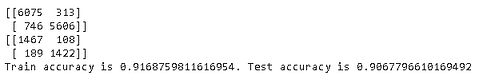The first and second matrices are confusion matrices on the train and test set, respectively. The confusion matrix goes deeper than classification accuracy by showing the correct and incorrect (i.e. true or false) predictions on each class.

Let’s first focus on the accuracy and then dive deep into the confusion matrix and related metrics.

One way to improve the performance of a model is to search for optimal hyperparameters. Adjusting the hyperparameters is like tuning the model. There are many hyperparameters of the random forest but the most important ones are the number of trees (n_estimators) and the maximum depth of an individual tree (max_depth).

We will use the GridSearchCV class of scikit-learn. It allows selecting the best parameters from a range of values. Let’s first create a dictionary that includes a set of values for n_estimators and max_depth. I will select the values around the ones we used previously.
```parameters = {'max_depth':[8,10,12,14],
'n_estimators':[175,200,225,250]}```

You can try more values or hyperparameters. There is not a single correct answer. We can now pass this dictionary to a GridSearchCV object along with an estimator.
```rf = RandomForestClassifier()
gridsearch = GridSearchCV(rf, param_grid=parameters, cv=5)
gridsearch.fit(X_train_selected, y_train)```

The cv parameter is doing the cross-validation.

We have trained the GridSearchCV object. Let’s see what the best parameters are:
```gridsearch.best_params_
{'max_depth': 12, 'n_estimators': 225}```

I have run the GridSearchCV one more time with values around 12 and 225. The best parameters turned out to be 13 and 235.

Let’s see the confusion matrix and accuracy with these new hyperparameter values.
```rf = RandomForestClassifier(max_depth=13, n_estimators=235)
rf.fit(X_train_selected, y_train)
y_pred = rf.predict(X_train_selected)
cm_train = confusion_matrix(y_train, y_pred)
print(cm_train)
y_test_pred = rf.predict(X_test_selected)
cm_test = confusion_matrix(y_test, y_test_pred)
print(cm_test)
train_acc = (cm_train + cm_train) / cm_train.sum()
test_acc = (cm_test + cm_test) / cm_test.sum()
print(f'Train accuracy is {train_acc}. Test accuracy is {test_acc}')```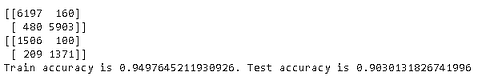The accuracy on the training set increased but we did not achieve anything on the test set. If we can collect more data which is usually the best way to increase the accuracy, test accuracy might also improve with these new parameters.

If you recall from the previous post, we had eliminated the 4 features which are less informative compared to other ones. In some cases, it is a good practice to eliminate less informative or uncorrelated features not to put unnecessary computation burden on the model. However, these eliminated features might slightly improve the accuracy so it comes down to a decision between performance enhancement and computation burden.

I played around with the hyperparameter values and trained with all the features. Here is the result: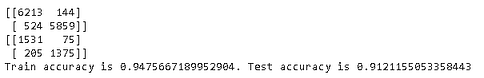We have achieved an approximately %1 increase in test accuracy which is also an improvement in terms of overfitting.

Our task is to predict if a customer will churn (i.e. stop being a customer). Thus, the focus should be on the positive class (1). We have to predict all the positive classes (Exited=1) correctly. We can afford to have some wrong predictions on the negative class (Exited=0).

We need to take the accuracy one step further. Let’s start with the confusion matrix.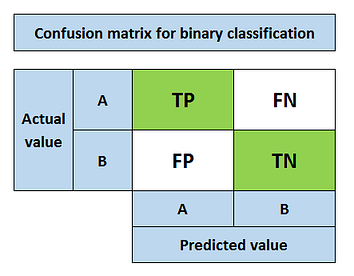(Image by author)
• True positive (TP): Predicting positive class as positive (ok)
• False positive (FP): Predicting negative class as positive (not ok)
• False negative (FN): Predicting positive class as negative (not ok)
• True negative (TN): Predicting negative class as negative (ok)

Since we want to predict customer churn as much as possible, we aim to maximize TP and minimize FN.

FN occurs when we predict “the customer will not churn (0)” but, in the actual situation, customer churns.

It is time to introduce two metrics which are precision and recall.

Precision
measures how good our model is when the prediction is positive.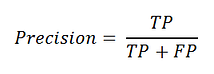The focus of precision is positive predictions. It indicates how many positive predictions are true.

Recall
measures how good our model is at correctly predicting positive classes.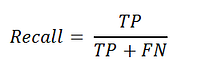The focus of recall is actual positive classes. It indicates how many of the positive classes the model is able to predict correctly.

We want to predict all the positive classes so recall is the appropriate metric for our task. Maximizing TP and/or minimizing FN will increase the recall value.

Here are the confusion matrices on train and test sets: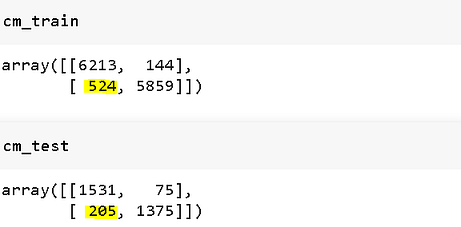We need to minimize the values marked with yellow which are false negatives (FN).

One way to achieve this is to tell the model that “positive class (1) is more important than the negative class (0)”. With our random forest classifier, it can be achieved by the class_weight parameter.
```rf = RandomForestClassifier(max_depth=12, n_estimators=245,
class_weight={0:1, 1:3})
rf.fit(X_train_transformed, y_train)```

We passed a dictionary that contains weights for each class. I set it as 3 to 1 as an example.

Here are the new confusion matrices: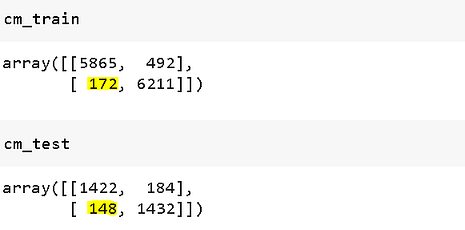The number of false positives is greatly reduced. The wrong predictions on the positive class are more penalized than the ones on negative class. Thus, the model leans towards making mistakes on the positive class as low as possible.

There is a downside to this approach. While getting better at predicting the positive class, the overall accuracy might get worse. Let’s check.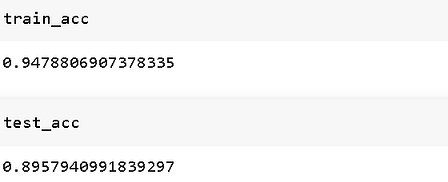The accuracy on the test set went down to %89.57 from %91.21. Thus, it comes down to a business decision. If we just want to predict all positive classes and do not care about the overall accuracy, we can further increase the weight of the positive class.

For instance, here is the confusion matrices and accuracy when we assign the weights as 10 to 1: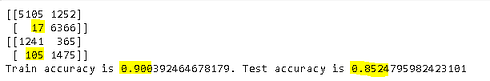We can also try different algorithms and see if the performance gets better. However, more complex models need more data. They are data-hungry. Gradient boosted decision tree (GBDT) and its variations (e.g. XGBOOST, LightGBM) can also be tried but I think there can only be a slight increase in the performance.

When the complexity of the task and the amount of data are considered, I think random forests will do the job just fine.

Thank you for reading. Please let me know if you have any feedback.

“Improving the Performance of a Machine Learning Model”
– Soner Yıldırım

### Related Stories

Jan 06, 2023

#### Top 2 Online Data Science Courses to Improve your Career in 2023

The discipline of Data Science is expanding quickly and has enormous promise. It is used in various sectors, including manufacturing, retail, healt...By Nikos_DataSource
Nov 25, 2021

#### 5 Tips To Ace Your Job Interview For A Data Scientist Opening

5 Tips To Ace Your Job Interview For A Data Scientist Opening.PNG 795.94 KBImage SourceAspiring data scientists have a bright future ahead of them....By Daniel Morales
Nov 12, 2021

#### When to Avoid Deep Learning